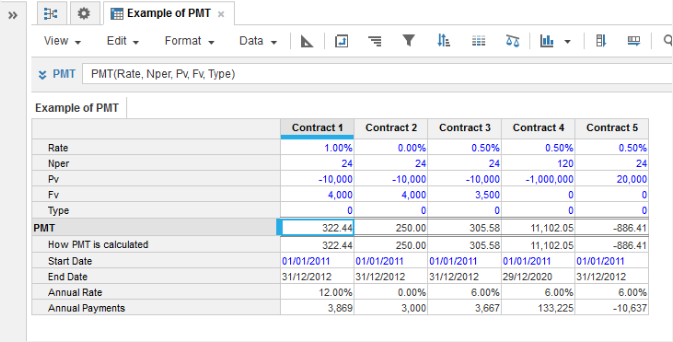1. Calculation functions
2. All Functions
3. Financial Functions
4. PMTCalculates the payments for a loan or annuity with constant payments and a constant interest rate.

PMT Formula:

PMT=
IF Rate = 0 THEN
(-Pv -Fv) / Nper
ELSE
(Fv + Pv * (1 + Rate) Nper) * Rate / ((1 - (1 + Rate) Nper )* (1 + Rate * Type))

where Type is either 0 for payments at the end of each period, 1 for payments at the beginning of each period.

## Syntax

PMT(r, n, p, [f], [t])

where:

• r: Number: Interest rate per period
• n: Number: The number of periods
• p: Number: Present value or the initial investment. Cash outflows such as investments are entered as negative numbers. If you are taking out a loan, this is a cash inflow so is entered as a positive number
• f: Number: future or residual value (optional)
• v: Number: when the payments are made. 0 or omitted means payments are made at the end of each period, non-zero means payments are made at the start of each period (optional)

## Format

Input Format Output Format

r: Rate: Number (percentage)

n: Nper: Number

p: Pv: Number

f: Fv: Number

v: Type: Number (binary)

Number

## Arguments

The function uses the following arguments:

• r: Numeric line item, property, or expression - percentage
• n: Numeric line item, property, or expression
• p: Numeric line item, property, or expression
• [f]: Numeric line item, property, or expression (optional)
• [t]: Numeric line item, property, or expression - binary (optional)

## Constraints

The function has the following constraints:

• Cash outflows are entered as negative numbers, cash inflows as positive numbers.

## Example

Contract 1 represents a cash investment Pv of 10000 at the end of period 1. The duration of the investment Nper is 24 months at which point the residual value Fv of 4000 is returned as a lump sum. The monthly interest rate Rate is 1.0% per period (approx 12.0 % per annum).

PMT calculates the 24 equal monthly payments of 322.44 to achieve this rate of interest.

PMT(Rate, Nper, Pv, Fv, Type)Contract 5 represents a loan of 20000. With an interest rate of 0.5% per period, 24 equal periodic payments of 886.41 are needed.